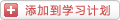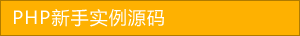﻿ PHP排序算法之快速排序(Quick Sort)及其优化算法详解 - php应用 - PHP粉丝网

# PHP排序算法之快速排序(Quick Sort)及其优化算法详解

##### 发布:smiling　来源: PHP粉丝网 　添加日期：2021-09-11 11:08:27　浏览: 评论：0

6   2   7   3   8   9

\$low = 0;

\$high = 5;

\$pivot = 6;

3   2   7   6   8   9

1. //这时候,\$high 减小为 3
2. \$low = 0;
3. \$high = 3;
4. \$pivot = 6;

3   2   6   7   8   9

1. //这时候,\$high 减小为 3
2. \$low = 2;
3. \$high = 3;
4. \$pivot = 6;

3   2   6   7   8   9

1. //这时候,\$high 减小为 3
2. \$low = 2;
3. \$high = 2;
4. \$pivot = 6;

1. //交换函数
2. function swap(array &\$arr,\$a,\$b){
3.   \$temp = \$arr[\$a];
4.   \$arr[\$a] = \$arr[\$b];
5.   \$arr[\$b] = \$temp
6. //主函数：
7. function QuickSort(array &\$arr){
8.   \$low = 0;
9.   \$high = count(\$arr) - 1;
10.   QSort(\$arr,\$low,\$high);

1. function QSort(array &\$arr,\$low,\$high){
2.   //当 \$low >= \$high 时表示不能再进行分组，已经能够得出正确结果了
3.   if(\$low < \$high){
4.     \$pivot = Partition(\$arr,\$low,\$high); //将\$arr[\$low...\$high]一分为二，算出枢轴值
5.     QSort(\$arr,\$low,\$pivot - 1); //对低子表（\$pivot左边的记录）进行递归排序
6.     QSort(\$arr,\$pivot + 1,\$high); //对高子表（\$pivot右边的记录）进行递归排序
7.   }

1. //选取数组当中的一个关键字，使得它处于数组某个位置时，左边的值比它小，右边的值比它大，该关键字叫做枢轴
2. //使枢轴记录到位，并返回其所在位置
3. function Partition(array &\$arr,\$low,\$high){
4.   \$pivot = \$arr[\$low];  //选取子数组第一个元素作为枢轴
5.   while(\$low < \$high){ //从数组的两端交替向中间扫描（当 \$low 和 \$high 碰头时结束循环）
6.     while(\$low < \$high && \$arr[\$high] >= \$pivot){
7.       \$high --;
8.     }
9.     swap(\$arr,\$low,\$high); //终于遇到一个比\$pivot小的数，将其放到数组低端
10.     while(\$low < \$high && \$arr[\$low] <= \$pivot){
11.       \$low ++;
12.     }
13.     swap(\$arr,\$low,\$high); //终于遇到一个比\$pivot大的数，将其放到数组高端
14.   }
15.   return \$low;  //返回high也行，毕竟最后low和high都是停留在pivot下标处

1. function swap(array &\$arr,\$a,\$b){
2.   \$temp = \$arr[\$a];
3.   \$arr[\$a] = \$arr[\$b];
4.   \$arr[\$b] = \$temp
5. function Partition(array &\$arr,\$low,\$high){
6.   \$pivot = \$arr[\$low];  //选取子数组第一个元素作为枢轴
7.   while(\$low < \$high){ //从数组的两端交替向中间扫描
8.     while(\$low < \$high && \$arr[\$high] >= \$pivot){
9.       \$high --;
10.     }
11.     swap(\$arr,\$low,\$high); //终于遇到一个比\$pivot小的数，将其放到数组低端
12.     while(\$low < \$high && \$arr[\$low] <= \$pivot){
13.       \$low ++;
14.     }
15.     swap(\$arr,\$low,\$high); //终于遇到一个比\$pivot大的数，将其放到数组高端
16.   }
17.   return \$low;  //返回high也行，毕竟最后low和high都是停留在pivot下标处
18. function QSort(array &\$arr,\$low,\$high){
19.   if(\$low < \$high){
20.     \$pivot = Partition(\$arr,\$low,\$high); //将\$arr[\$low...\$high]一分为二，算出枢轴值
21.     QSort(\$arr,\$low,\$pivot - 1);  //对低子表进行递归排序
22.     QSort(\$arr,\$pivot + 1,\$high); //对高子表进行递归排序
23.   }
24. function QuickSort(array &\$arr){
25.   \$low = 0;
26.   \$high = count(\$arr) - 1;
27.   QSort(\$arr,\$low,\$high);

1. \$arr = array(9,1,5,8,3,7,4,6,2);
2. QuickSort(\$arr);
3. var_dump(\$arr);

1. array(9) {
2.  =>
3.  int(1)
4.  =>
5.  int(2)
6.  =>
7.  int(3)
8.  =>
9.  int(4)
10.  =>
11.  int(5)
12.  =>
13.  int(6)
14.  =>
15.  int(7)
16.  =>
17.  int(8)
18.  =>
19.  int(9)

9   1   5   8   3   7   4   6   2

1、随机选取：随机选取 \$low 到 \$high 之间的数值，但是这样的做法有些撞大运的感觉了，万一没撞成功呢，那上面的问题还是没有解决。

2、三数取中法：取三个关键字先进行排序，取出中间数作为枢轴。这三个数一般取最左端、最右端和中间三个数，也可以随机取三个数。这样的取法得到的枢轴为中间数的可能性就大大提高了。由于整个序列是无序的，随机选择三个数和从左中右端取出三个数其实就是同一回事。而且随机数生成器本身还会带来时间的开销，因此随机生成不予考虑。

1. function Partition(array &\$arr,\$low,\$high){
2.   \$mid = floor(\$low + (\$high - \$low) / 2);  //计算数组中间的元素的下标
3.   if(\$arr[\$low] > \$arr[\$high]){
4.     swap(\$arr,\$low,\$high);
5.   }
6.   if(\$arr[\$mid] > \$arr[\$high]){
7.     swap(\$arr,\$mid,\$high);
8.   }
9.   if(\$arr[\$low] < \$arr[\$mid]){
10.     swap(\$arr,\$low,\$mid);
11.   }
12.   //经过上面三步之后，\$arr[\$low]已经成为整个序列左中右端三个关键字的中间值
13.   \$pivot = \$arr[\$low];
14.   while(\$low < \$high){  //从数组的两端交替向中间扫描（当 \$low 和 \$high 碰头时结束循环）
15.     while(\$low < \$high && \$arr[\$high] >= \$pivot){
16.       \$high --;
17.     }
18.     swap(\$arr,\$low,\$high); //终于遇到一个比\$pivot小的数，将其放到数组低端
19.     while(\$low < \$high && \$arr[\$low] <= \$pivot){
20.       \$low ++;
21.     }
22.     swap(\$arr,\$low,\$high); //终于遇到一个比\$pivot大的数，将其放到数组高端
23.   }
24.   return \$low;  //返回high也行，毕竟最后low和high都是停留在pivot下标处

5   1   9   3   7   4   8   6   2

1. function Partition(array &\$arr,\$low,\$high){
2.   \$mid = floor(\$low + (\$high - \$low) / 2);  //计算数组中间的元素的下标
3.   if(\$arr[\$low] > \$arr[\$high]){
4.     swap(\$arr,\$low,\$high);
5.   }
6.   if(\$arr[\$mid] > \$arr[\$high]){
7.     swap(\$arr,\$mid,\$high);
8.   }
9.   if(\$arr[\$low] < \$arr[\$mid]){
10.     swap(\$arr,\$low,\$mid);
11.   }
12.   //经过上面三步之后，\$arr[\$low]已经成为整个序列左中右端三个关键字的中间值
13.   \$pivot = \$arr[\$low];
14.   \$temp = \$pivot
15.   while(\$low < \$high){  //从数组的两端交替向中间扫描（当 \$low 和 \$high 碰头时结束循环）
16.     while(\$low < \$high && \$arr[\$high] >= \$pivot){
17.       \$high --;
18.     }
19.     //swap(\$arr,\$low,\$high); //终于遇到一个比\$pivot小的数，将其放到数组低端
20.     \$arr[\$low] = \$arr[\$high];  //使用替换而不是交换的方式进行操作
21.     while(\$low < \$high && \$arr[\$low] <= \$pivot){
22.       \$low ++;
23.     }
24.     //swap(\$arr,\$low,\$high); //终于遇到一个比\$pivot大的数，将其放到数组高端
25.     \$arr[\$high] = \$arr[\$low];
26.   }
27.   \$arr[\$low] = \$temp;  //将枢轴数值替换回 \$arr[\$low];
28.   return \$low;  //返回high也行，毕竟最后low和high都是停留在pivot下标处

1. //规定数组长度阀值
2. #define MAX_LENGTH_INSERT_SORT 7
3. function QSort(array &\$arr,\$low,\$high){
4.   //当 \$low >= \$high 时表示不能再进行分组，已经能够得出正确结果了
5.   if((\$high - \$low) > MAX_LENGTH_INSERT_SORT){
6.     \$pivot = Partition(\$arr,\$low,\$high); //将\$arr[\$low...\$high]一分为二，算出枢轴值
7.     QSort(\$arr,\$low,\$pivot - 1); //对低子表（\$pivot左边的记录）进行递归排序
8.     QSort(\$arr,\$pivot + 1,\$high); //对高子表（\$pivot右边的记录）进行递归排序
9.   }else
10.     //直接插入排序
11.     InsertSort(\$arr);
12.   }

PS：上面的直接插入排序算法大家可以参考：《PHP排序算法之直接插入排序（Straight Insertion Sort）》

1. //规定数组长度阀值
2. #define MAX_LENGTH_INSERT_SORT 7
3. function QSort(array &\$arr,\$low,\$high){
4.   //当 \$low >= \$high 时表示不能再进行分组，已经能够得出正确结果了
5.   if((\$high - \$low) > MAX_LENGTH_INSERT_SORT){
6.     while(\$low < \$high){
7.       \$pivot = Partition(\$arr,\$low,\$high); //将\$arr[\$low...\$high]一分为二，算出枢轴值
8.       QSort(\$arr,\$low,\$pivot - 1); //对低子表（\$pivot左边的记录）进行递归排序
9.       \$low = \$pivot + 1;
10.     }
11.   }else
12.     //直接插入排序
13.     InsertSort(\$arr);
14.   }

### 分享到： document.write('<a href="http://v.t.sina.com.cn/share/share.php?url='+encodeURIComponent(location.href)+'&appkey=3172366919&title='+encodeURIComponent('PHP排序算法之快速排序(Quick Sort)及其优化算法详解')+'" title="分享到新浪微博" class="t1" target="_blank">&nbsp;</a>'); document.write('<a href="http://www.douban.com/recommend/?url='+encodeURIComponent(location.href)+'&title='+encodeURIComponent('PHP排序算法之快速排序(Quick Sort)及其优化算法详解')+'" title="分享到豆瓣" class="t2" target="_blank">&nbsp;</a>'); document.write('<a href="http://share.renren.com/share/buttonshare.do?link='+encodeURIComponent(location.href)+'&title='+encodeURIComponent('PHP排序算法之快速排序(Quick Sort)及其优化算法详解')+'" title="分享到人人" class="t3" target="_blank">&nbsp;</a>'); document.write('<a href="http://www.kaixin001.com/repaste/share.php?rtitle='+encodeURIComponent('PHP排序算法之快速排序(Quick Sort)及其优化算法详解')+'&rurl='+encodeURIComponent(location.href)+'&rcontent=" title="分享到开心网" class="t4" target="_blank">&nbsp;</a>'); document.write('<a href="http://sns.qzone.qq.com/cgi-bin/qzshare/cgi_qzshare_onekey?url='+encodeURIComponent(location.href)+'" title="分享到QQ空间" class="t5" target="_blank">&nbsp;</a>');